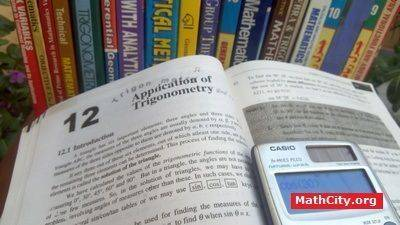# Chapter 12: Application of TrigonometryNotes (Solutions) of Chapter 12: Application of Trigonometry, Text Book of Algebra and Trigonometry Class XI (Mathematics FSc Part 1 or HSSC-I), Punjab Text Book Board, Lahore.

• Introduction
• Tables of Trigonometric Ratios
• Exercise 12.1
• Solution of Right Triangles
• Exercise 12.2
• Heights and Distances
• Engineering and Heights and Distances
• Exercise 12.3
• Oblique Triangles
• The Law of Cosine
• The Law of Sines
• The Law of Tangents
• Half Angle Formulas
• Solution of Oblique Triangles
• Case I : When measure of one side and two angles are given
• Exercise 12.4
• Case II : When measure of two sides and their included angle are given
• Exercise 12.5
• Case III : When measures of three sides are given
• Exercise 12.6
• Area of Triangle
• Exercise 12.7
• Circles Connected with Triangle
• Circum-Circle
• In-Circle
• Escribed Circles
• Engineering and Circles Connected with Triangles
• Exercise 12.8

The following short questions was send by Mr. Akhtar Abbas.

• fsc/fsc_part_1_solutions/ch12